The previous post describes how to plot a basic bubble plot. This post aims to show how you can customize the features of your basic bubble plot with several examples.

## Color

After plotting a basic bubble plot with the `scatter()` function of matplotlib, you can customize it by changing the color of the markers. You can use the color parameter `c` for this purpose.

``````# libraries
import matplotlib.pyplot as plt
import numpy as np

# create data
x = np.random.rand(5)
y = np.random.rand(5)
z = np.random.rand(5)

# Change color with c and alpha
plt.scatter(x, y, s=z*4000, c="red", alpha=0.4)

# show the graph
plt.show()``````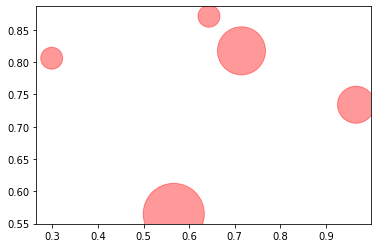## Shape

As you can change the color of the markers, it is also possible to change the shapes by giving `marker` parameter to the `scatter()` function.

``````# libraries
import matplotlib.pyplot as plt
import numpy as np

# create data
x = np.random.rand(5)
y = np.random.rand(5)
z = np.random.rand(5)

# Change shape with marker
plt.scatter(x, y, s=z*4000, marker="D")

# show the graph
plt.show()``````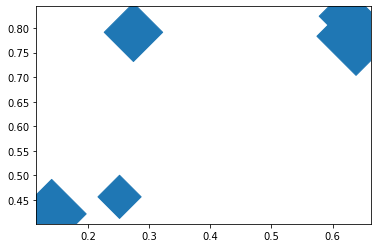## Global Size

In order to change the size of each marker, `s` size parameter can be used. In the example below, `s` parameter is set as a multiplier of z data points, so the sizes of the markers depends on the z values.

``````# libraries
import matplotlib.pyplot as plt
import numpy as np

# create data
x = np.random.rand(5)
y = np.random.rand(5)
z = np.random.rand(5)

# Change global size playing with s
plt.scatter(x, y, s=z*200)

# show the graph
plt.show()``````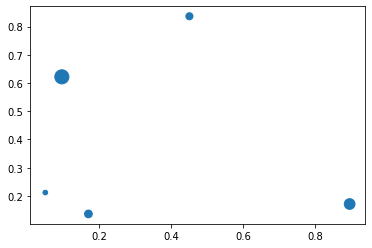## Edges

`linewidth` parameter is useful to set the edge thickness of the markers in a basic bubble plot built with matplotlib.

``````# libraries
import matplotlib.pyplot as plt
import numpy as np

# create data
x = np.random.rand(5)
y = np.random.rand(5)
z = np.random.rand(5)

# Change line around dot
plt.scatter(x, y, s=z*4000, c="green", alpha=0.4, linewidth=6)

# show the graph
plt.show()``````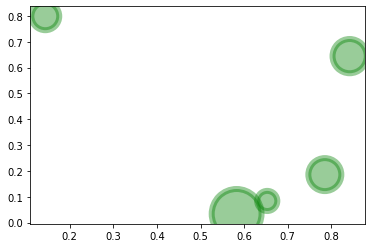## Seaborn Style

It is possible to benefit from seaborn library style when plotting charts in matplotlib. You just need to load the seaborn library and use seaborn `set_theme()` function!

``````# libraries
import matplotlib.pyplot as plt
import numpy as np

# create data
x = np.random.rand(5)
y = np.random.rand(5)
z = np.random.rand(5)

# pimp your plot with the seaborn style
import seaborn as sns
sns.set_theme()

# plot
plt.scatter(x, y, s=z*4000, c="green", alpha=0.4, linewidth=6)

# Add titles (main and on axis)
plt.xlabel("the X axis")
plt.ylabel("the Y axis")
plt.title("A bubble plot", loc="left")

# show the graph
plt.show()``````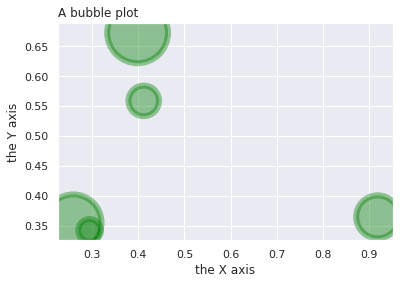## Contact & Edit

👋 This document is a work by Yan Holtz. Any feedback is highly encouraged. You can fill an issue on Github, drop me a message onTwitter, or send an email pasting `yan.holtz.data` with `gmail.com`.